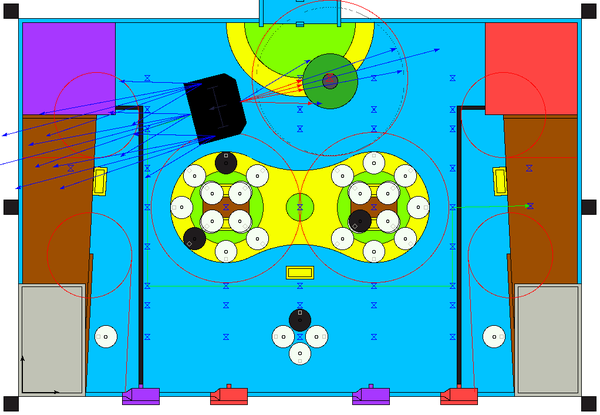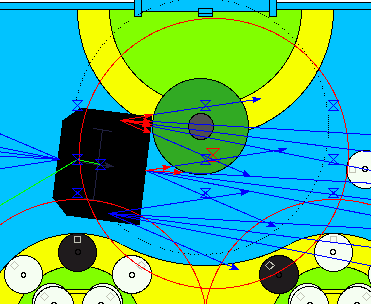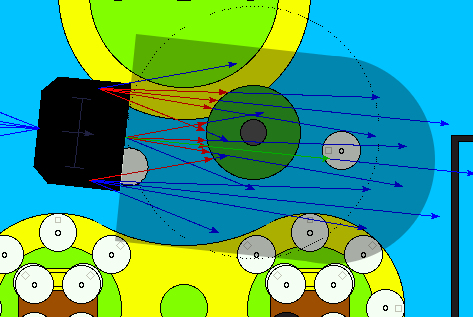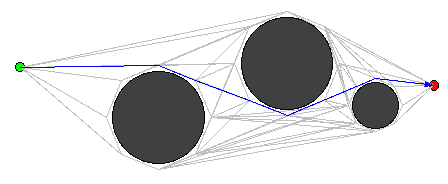# Path finding

An autonomous robot needs to be able to avoid obstacles, which may be static (table fixed elements) or dynamic (opponent robots).

Our robot uses the A* algorithm to find its path. This algorithm is an optimisation of the Dijkstra'as algorithm using an heuristic to prefer nodes which are in the expected direction. Those algorithms find the shortest path between two nodes in a graph, please refer to the Wikipedia articles for details.

As we are using microcontrollers with limited CPU power and memory, we try to reduce the number of nodes in the graph given to the algorithm. Here is an example for the 2012 robot:Blue markers are the path finding nodes. There are fixed nodes in a grid pattern, fixed nodes at some interesting positions (in unload holds for example), and two extra nodes, one for the current robot position and one for the desired destination. Every nodes are given a node identifier.

Graph arcs (connexions between nodes, associated with a weight) are not stored in our program, but rather recomputed at every path finding iteration (see the the neighbor callback functions in path.c).

When an arc needs to be evaluated, the `path_blocking` function in path.c tests if there is an obstacle which forbid robot movement. Obstacles are drawn in red in the above image. They are made really large to take our robot dimension into account as the algorithm considers our robot is a single point. If there is no blocking obstacle, the distance between the two nodes is used as the arc weight.

Here is an example path. Green arrow is the found path, blue arrows are the US sensor rays, and the green circle represents an opponent robot.Sometime, the robot is really near an obstacle, and therefore, any path will be impossible as each arcs will intersect with the obstacle. In this case, we use a escape mode, in which the robot will try to escape from the obstacle using the shortest path (the arcs from the source node see their weight multiplied by 8). In the escape mode, the robot will try to limit the rotations in order not to touch the opponent robot. In this example, the robot will go backward to escape the obstacle before continuing its path:## Obstacle avoidance

Once the path is being followed by the robot, the US sensor (and hopefully in a near future, the beacon system) reports obstacles positions. Invalid positions (out of the table for example) are ignored. If an obstacle is found on the robot path, it stops and computes a new path.

To do this, a zone is defined around the path to the next destination. If there is a obstacle in this zone, it is considered blocking.## Implementation

The path/astar module contains the A* algorithm implementation, reused every years (it also contain a Dijkstra algorithm used for strategy evaluation, see the to be written article about strategy).

The path.c file contains specific definitions (neighbor callbacks, node definitions, blocking computations), and move.c FSM will follow the computed path. You can also find 2011 version and 2010 version.

Obstacle detection on the path is done in radar.c.

## Previous implementation

We introduced the use of A* algorithm and the dynamic computation of neighbor in 2010, because of the large number of obstacles on the table. Previous algorithm was using a large in memory graph with a connexion between every possible nodes. The node list was dynamically generated around obstacles, you can still find this algorithm in the path module.